## Geometry Through the Eyes of Felix Klein

### Geometry Through the Eyes of Felix Klein

Even with the benefit of enormous hindsight, it is hard to introduce complex numbers in a compelling manner. Historically, we have seen how cubic equations forced them upon us algebraically, and in discussing Cotes’ work we saw something of the inevitability of their geometric interpretation. In this section we will attempt to show how complex numbers arise very naturally, almost inevitably, from a careful re-examination of plane Euclidean geometry.

[…]

Although the ancient Greeks made many beautiful and remarkable discoveries in geometry, it was two thousand years later that Felix Klein first asked and answered the question, “What is geometry?”

Let us restrict ourselves from the outset to plane geometry. One might begin by saying that this is the study of geometric properties of geometric figures in the plane, but what are (i) “geometric properties”, and (ii) “geometric figures”? We will concentrate on (i), swiftly passing over (ii) by interpreting “geometric figure” as anything we might choose to draw on an infinitely large piece of flat paper with an infinitely fine pen.

As for (i), we begin by noting that if two figures (e.g., two triangles), have the same geometric properties, then (from the point of view of geometry) they must be the “same”, “equal”, or, as one usually says, congruent. Thus if we had a clear definition of congruence (“geometric equality”) then we could reverse this observation and define geometric properties as those properties that are common to all congruent figures. How, then, can we tell if two figures are geometrically equal?

Consider the triangles in [fig 23], and imagine that they are pieces of paper you could pick up in your hand. To see if T is congruent with T’, you could pick up T and check whether it could be placed on top of T’. Note that it is essential that we be allowed to move in space: in order to place T on top of T˜ we must first flip it over; we can’t just slide T around within the plane. Tentatively generalizing, this suggests that a figure F is congruent to another figure F’ if there exists a motion of F through space that makes it coincide with F’. Note that the discussion suggests that there are two fundamentally different types of motion: those that involve flipping the figure over, and those that do no. Later, we shall return to this important point.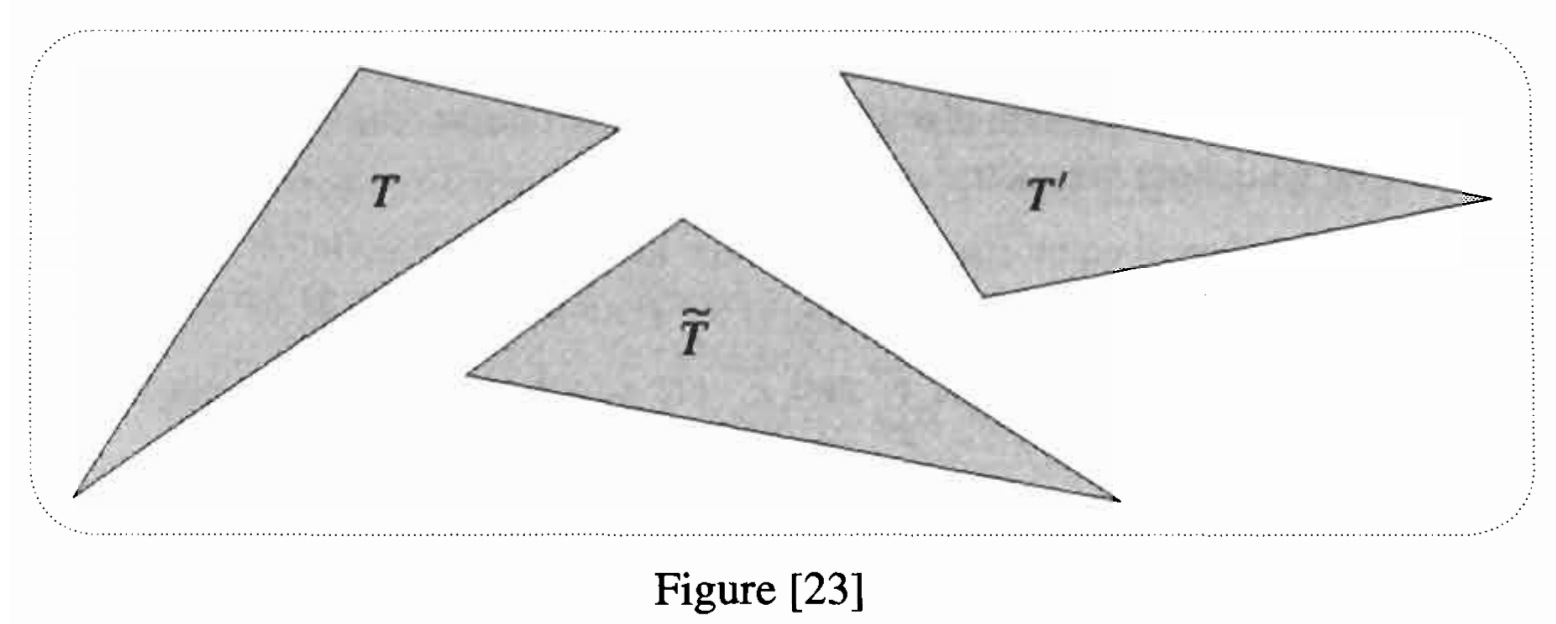It is clearly somewhat unsatisfactory that in attempting to define geometry in the plane we have appealed to the idea of motion through space. We now rectify this. Returning to [fig 23], imagine that T and T’ are drawn on separate, transparent sheets of plastic. Instead of picking up just the triangle T, we now pick up the entire sheet on which it is drawn, then try to place it on the second sheet so as to make T coincide with T’. At the end of this motion, each point A on T‘s sheet lies over a point A’ of T’‘s sheet, and we can now define the motion M to be this mapping AA’ = M(A) of the plane to itself.

However, not any old mapping qualifies as a motion, for we must also capture the (previously implicit) idea of the sheet remaining rigid while it moves, so that distances between points remain constant during the motion. Here, then, is our definition:

A motion M is a mapping of the plane to itself such that the distance between any two points A and B is equal to the distance between their images A’ = M(A) and B’ = M(B). (22)

Note that what we have called a motion is often termed a “rigid motion”, or an “isometry”.

Armed with this precise concept of motion, our final definition of geometric equality becomes:

F is congruent to F’, written F ≅ F’, if there exists a motion M such that F’ = M(F). (23)

Next, as a consequence of our earlier discussion, a geometric property of a figure is one that is unaltered by all possible motions of the figure. Finally, in answer to the opening question of “What is geometry?”, Klein would answer that it is the study of these so-called invariants of the set of motions.

One of the most remarkable discoveries of the last century was that Euclidean geometry is not the only possible geometry. Two of these so-called non-Euclidean geometries will be studied in Chapter 6, but for the moment we wish only to explain how Klein was able to generalize the above ideas so as to embrace such new geometries.

The aim in (23) was to use a family of transformations to introduce the concept of geometric equality. But will this ≅-type of equality behave in the way we would like and expect? To answer this we must first make these expectations explicit. So as not to confuse this general discussion with the particular concept of congruence in (23), let us denote geometric equality by ~.

(i) A figure should equal itself: F ~ F’, for all F.

(ii) If F equals F’, then F’ should equal F: F ~ F’F’ ~ F.

(iii) If F and F’ are equal, and F’ and F” are equal, then F and F” should also be equal: F ~ F’ & F’ ~ F”F ~ F”.

Any relation satisfying these expectations is called an equivalence relation.

Now suppose that we retain the definition (23) of geometric equality, but that we generalize the definition of “motion” given in (22) by replacing the family of distance-preserving transformations with some other family G of transformations.

It should be clear that not any old G will be compatible with our aim of defining geometric equality. Indeed, (i), (ii), and (iii) imply that G must have the following very special structure, which is illustrated in [fig 24].

(i) The family G must contain a transformation ε (called identity) that maps each point to itself.

(ii) If G contains a transformation M, then it must also contain a transformation M-1 (called the inverse) that undoes M. [Check for yourself that for M-1 to exist (let alone be a member of G) M must have the special properties of being (a) onto and (b) one-to-one, i.e., (a) every point must be the image of some point, and (b) distinct points must have distinct images.]

(iii) If and N are members of G then so is the composite transformation N ∘ M = (followed by N). This property of G is called closure.

We have thus arrived, very naturally, at a concept of fundamental importance in the whole of mathematics: a family G of transformations that satisfies these three requirements is called a group.Let us check that the motions defined in (22) do indeed form a group: (i) Since the identity transformation preserves distances, it is a motion. (ii) Provided it exists, the inverse of a motion will preserve distances and hence will be a motion itself. As for existence, (a) it is certainly plausible that when we apply a motion to the entire plane then the image is the entire plane — we will prove this later — and (b) the non-zero distance between distinct points is preserved by a motion, so their images are again distinct. (iii) If two transformations do not alter distances, then applying them in succession will not alter distances either, so the composition of two motions is another motion.

Klein’s idea was that we could first select a group G at will, then define a corresponding “geometry” as the study of the invariants of that G. [Klein first announced this idea in 1872 — when he was 23 years old! — at the University of Erlangen, and it has thus come to be known as his Erlangen Program.] For example, if we choose G to be the group of motions, we recover the familiar Euclidean geometry of the plane. But this is far from being the only geometry of the plane, as the so-called projective geometry of [fig 24] illustrates.

Klein’s vision of geometry was broader still. We have been concerned with what geometries are possible when figures are drawn anywhere in the plane, but suppose for example that we are only allowed to draw within some disc D. It should be clear that we can construct “geometries of D” in exactly the same way that we constructed geometries of the plane: given a group H of transformations of D to itself, the corresponding geometry is the study of the invariants of H. If you doubt that any such group exists, consider the set of all rotations around the center of D.

The reader may well feel that the above discussion is a chronic case of mathematical generalization running amuck — that the resulting conception of geometry is (to coin a phrase) “as subtle as it is useless”. Nothing could be further from the truth! In Chapter 3 we shall be led, very naturally, to consider a particularly interesting group of transformations of a disc to itself. The resulting non-Euclidean geometry is called hyperbolic or Lobachevskian geometry, and it is the subject of Chapter 6. Far from being useless, this geometry has proved to be an immensely powerful tool in diverse areas of mathematics, and the insights it continues to provide lie on the cutting edge of contemporary research.

 The excellent book by Nikulin and Shafarevich  is the only other work we know of in which a similar attempt is made.

 Here G is the group of projections. If we do a perspective drawing of figures in the plane, then the mapping from the plane to the “canvas” plane is called a perspectivity. A projection is then defined to be any sequence of perspectivities. Can you see why the set of projections should form a group?

 In more abstract settings it is necessary to add a fourth requirement of associativity, namely, A∘ (B∘ C) = (A∘ B)∘ C. Of course for transformations this is automatically true.

Note: QRI‘s Symmetry Theory of Valence (STV) refers to the claim that valence (the pleasure-pain axis) manifests in the symmetry of the mathematical object that corresponds to each experience such that the mathematical features of that object are isomorphic to the phenomenal character of that experience. Using the lens of Klein’s conception of geometry, one could in turn give to STV a strictly geometrical interpretation. Namely, that the shape of one’s experience will be of high valence when it contains geometric invariants. In addition, Noether’s theorem (one of QRI’s lineages, which states that “every differentiable symmetry of the action of a physical system has a corresponding conservation law”) along with physicalism of consciousness suggests that for every symmetry in the mathematical object that corresponds to consciousness there is a corresponding preserved quantity. Thus, one could posit– assuming that the STV is correct– high-valence states might be extremely energy-efficient in addition to feeling good.

We are currently preparing a paper that ties all of these threads together in a way that, we believe, may turn out to have tremendous explanatory power. In particular, this formal account of valence will be able to explain succinctly a wide range of disparate and exotic empirical phenomena such as:

1. Why phenomenal symmetry during psychedelic experiences is correlated with more extreme valence values.
2. How and why Jhanas present the way they do, namely, as having:
1. Extremely positive valence,
2. Profound phenomenal simplicity, and
3. Unusually high levels of energy-efficiency all at once.
3. The fact that moments of eternity are typically extremely blissful.
4. Why the phenomenal character of MDMA and 5-MeO-DMT manifest as both extremely symmetrical and high-valence.
5. Why intense regular stroboscopic stimulation has deep emotional effects.
6. And so much more…

Stay tuned!

Thanks to Alfredo Valverde de Loyola for pointing me to this excerpt in the book Visual Complex Analysis.

## Materializing Hyperbolic Spaces with Gradient-Index Optics and One-Way Mirrors

Burning Man is one week away, so I figured I would share a neat idea I’ve been hoarding  that could lead to a kick-ass Burning Man-style psychedelic art installation. If I have the time and resources to do so, I may even try to manifest this idea in real life at some point.

Around the time I was writing The Hyperbolic Geometry of DMT Experiences (cf. Eli5) I began asking myself how to help people develop a feel for what it is like to inhabit non-Euclidean phenomenal spaces. I later found out that Henry Segerman developed an immersive VR experience in which you can explore 3D hyperbolic spaces. That is fantastic, and a great step in the right direction. But I wanted to see if there was any way for us to experience 3D hyperbolic geometry in a material way without the aid of computers. Something that you could hold in your hand, like a sort of mystical amulet that works as a reminder of the vastness of the state-space of consciousness.

What I had in mind was along the lines of how we can, in a sense, visualize infinite (Euclidean) space using two parallel mirrors. I thought that maybe there could be a way to do the same but in a way that visualizes a hyperbolic space.

### One-Way Mirrors and 3D Space-Filling Shapes

Right now you can use one-way mirrors on the sides of a polyhedra whose edges are embedded with LEDs to create a fascinating “infinite space effect”:

This works perfectly for cubes in particular, given that cubes are symmetrical space-filling polyhedra. But as you can see in the video above, the effect is not quite perfect when we use dodecahedra (or any other Platonic solid). The corners simply do not align properly. This is because the solid angles of non-cube Platonic solids cannot be used to cover perfectly 4π steradians (i.e. what 8 cubes do when meeting at a corner):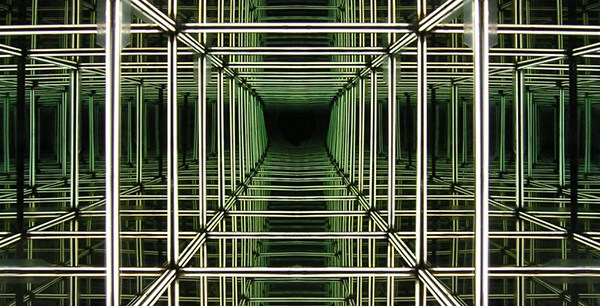This is not the case in hyperbolic space, though; arbitrary regular polyhedra can tesselate 3D hyperbolic spaces. For instance, one can use dodecahedra by choosing their size appropriately in such a way that they all have 90 degree angle corners (cf. Not Knot):

Perhaps, I thought to myself, there is a way to physically realize hyperbolic curvature and enable us to see what it is like to live in a place in which dodecahedra tesselate space. I kept thinking about this problem, and one day while riding the BART and introspecting on the geometry of sound, I realized that one could use gradient-index optics to create a solid in which light-paths behave as if the space was hyperbolic.

Gradient-index optics is the subfield of optics that specializes in the use of materials that have a smooth non-constant refractive index. One way to achieve this is to blend two transparent materials (e.g. two kinds of plastic) in such a way that the concentration of each type varies smoothly from one region to the next. As a consequence, light travels in unusual and bendy ways, like this:

### Materializing Hyperbolic Spaces

By carefully selecting various transparent plastics with different indices of refraction and blending them in a 3D printer in precisely the right proportions, one can in principle build solids in which the gradient-index properties of the end product instantiate a hyperbolic metric. If one were to place the material with the lowest refraction index at the very center in a dodecahedron and add materials of increasingly larger refractive indices all the way up to the corners, then the final effect could be one in which the dodecahedron has an interior in which light moves as if it were in a hyperbolic space. One can then place LED strips along the edges and seal the sides with one-way window film. Lo-and-behold, one would then quite literally be able to “hold infinity in the palm of your hand”: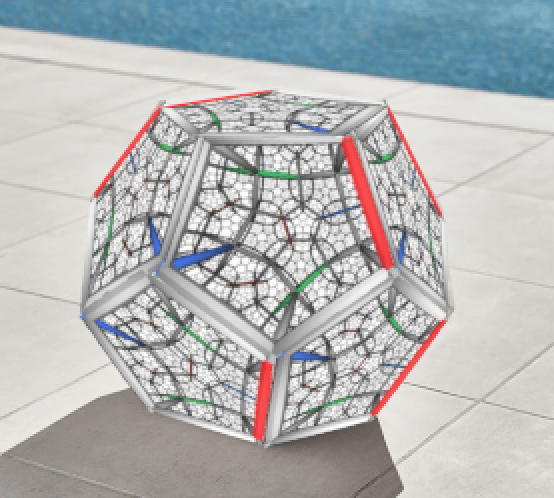I think that this sort of gadget would allow us to develop better intuitions for what the far-out (experiential) spaces people “visit” on psychedelics look like. One can then, in addition, generalize this to make space behave as if its 3D curvature was non-constant. One might even, perhaps, be able to visualize a black-hole by emulating its event-horizon using a region with extremely large refractive index.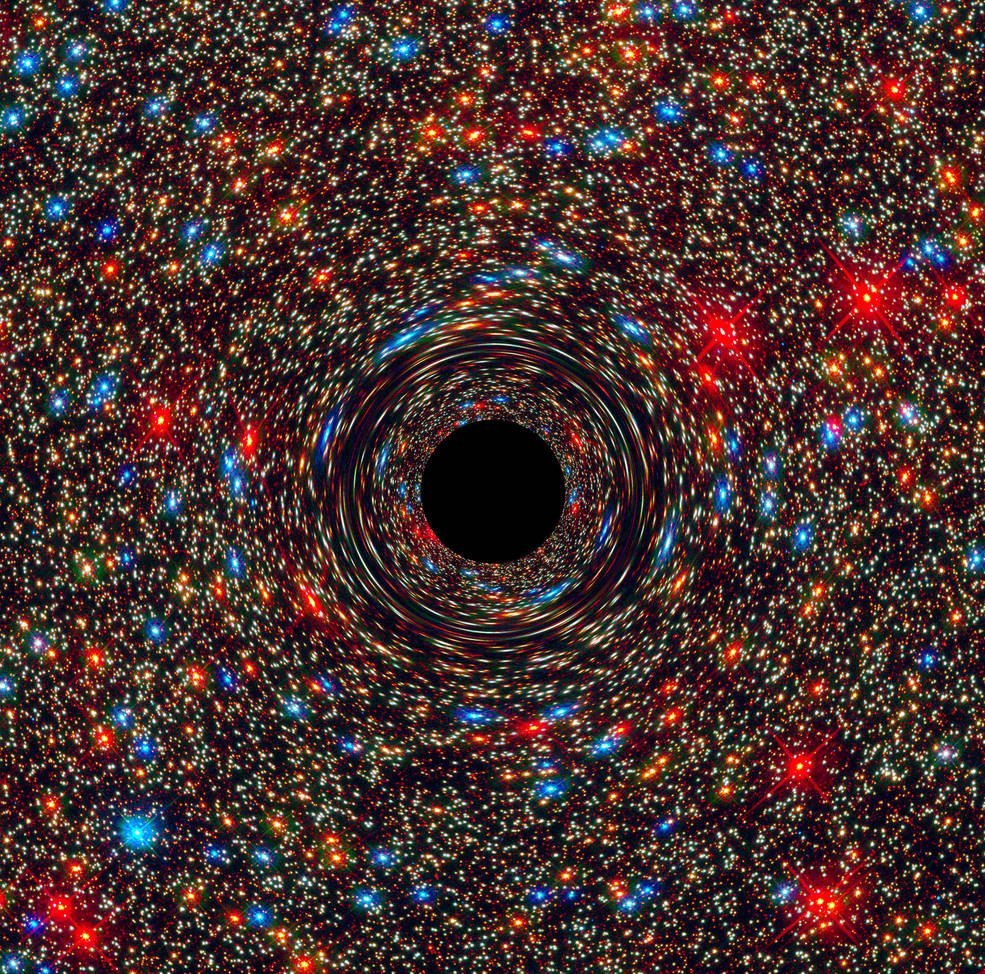### Challenges

I would like to conclude by considering some of the challenges that we would face trying to construct this. For instance, finding the right materials may be difficult because they would need to have a wide range of refractive indices, all be similarly transparent, able to smoothly blend with each other, and have low melting points. I am not a material scientist, but my gut feeling is that this is not currently impossible. Modern gradient-index optics already has a rather impressive level of precision.

Another challenge comes from the resolution of the 3D printer. Modern 3D printers have layers with a thickness between .2 to 0.025mm. It’s possible that this is simply not small enough to avoid visible discontinuities in the light-paths. At least in principle this could be surmounted by melting the last layer placed such that the new layer smoothly diffuses and partially blends with it in accordance with the desired hyperbolic metric.

An important caveat is that the medium in which we live (i.e. air at atmospheric pressure) is not very dense to begin with. In the example of the dodecahedra, this may represent a problem considering that the corners need to form 90 degree angles from the point of view of an outside observer. This would imply that the surrounding medium needs to have a higher refraction index than that of the transparent medium at the corners. This could be fixed by immersing the object in water or some other dense media (and designing it under the assumption of being surrounded by such a medium). Alternatively, one can simply fix the problem by using appropriately curved sides in lieu of straight planes. This may not be as aesthetically appealing, though, so it may pay off to brainstorm other clever approaches to deal with this that I haven’t thought of.

Above all, perhaps the most difficult challenge would be that of dealing with the inevitable presence of chromatic aberrations:

Since the degree to which a light-path bends in a medium depends on its frequency, how bendy light looks like with gradient-index optics is variable. If the LEDs placed at the edges of the polyhedra are white, we could expect very visible distortions and crazy rainbow patterns to emerge. This would perhaps be for the better when taken for its aesthetic value. But since the desired effect is one of actually materializing faithfully the behavior of light in hyperbolic space, this would be undesirable. The easiest way to deal with this problem would be to show the gadget in a darkened room and have only monochrome LEDs on the edges of the polyhedra whose frequency is tuned to the refractive gradient for which the metric is hyperbolic. More fancifully, it might be possible to overcome chromatic aberrations with the use of metamaterials (cf. “Metasurfaces enable improved optical lens performance“). Alas, my bedtime is approaching so I shall leave the nuts and bolts of this engineering challenge as an exercise for the reader…

## ELI5 “The Hyperbolic Geometry of DMT Experiences”##### I wrote the following in response to a comment on the r/RationalPsychonaut subreddit about this DMT article I wrote some time ago. The comment in question was: “Can somebody eli5 [explain like I am 5 years old] this for me?” So here is my attempt (more like “eli12”, but anyways):

In order to explain the core idea of the article I need to convey the main takeaways of the following four things:

1. Differential geometry,
2. How it relates to symmetry,
3. How it applies to experience, and
4. How the effects of DMT turn out to be explained (in part) by changes in the curvature of one’s experience of space (what we call “phenomenal space”).

### 1) Differential Geometry

If you are an ant on a ball, it may seem like you live on a “flat surface”. However, let’s imagine you do the following: You advance one centimeter in one direction, you turn 90 degrees and walk another centimeter, turn 90 degrees again and advance yet another centimeter. Logically, you just “traced three edges of a square” so you cannot be in the same place from which you departed. But let’s says that you somehow do happen to arrive at the same place. What happened? Well, it turns out the world in which you are walking is not quite flat! It’s very flat from your point of view, but overall it is a sphere! So you ARE able to walk along a triangle that happens to have three 90 degree corners.

That’s what we call a “positively curved space”. There the angles of triangles add up to more than 180 degrees. In flat spaces they add up to 180. And in “negatively curved spaces” (i.e. “negative Gaussian curvature” as talked about in the article) they add up to less than 180 degrees.Eight 90-degree triangles on the surface of a sphere

So let’s go back to the ant again. Now imagine that you are walking on some surface that, again, looks flat from your restricted point of view. You walk one centimeter, then turn 90 degrees, then walk another, turn 90 degrees, etc. for a total of, say, 5 times. And somehow you arrive at the same point! So now you traced a pentagon with 90 degree corners. How is that possible? The answer is that you are now in a “negatively curved space”, a kind of surface that in mathematics is called “hyperbolic”. Of course it sounds impossible that this could happen in real life. But the truth is that there are many hyperbolic surfaces that you can encounter in your daily life. Just to give an example, kale is a highly hyperbolic 2D surface (“H2” for short). It’s crumbly and very curved. So an ant might actually be able to walk along a regular pentagon with 90-degree corners if it’s walking on kale (cf. Too Many Triangles).An ant walking on kale may infer that the world is an H2 space.

In brief, hyperbolic geometry is the study of spaces that have this quality of negative curvature. Now, how is this related to symmetry?

### 2) How it relates to symmetry

As mentioned, on the surface of a sphere you can find triangles with 90 degree corners. In fact, you can partition the surface of a sphere into 8 regular triangles, each with 90 degree corners. Now, there are also other ways of partitioning the surface of a sphere with regular shapes (“regular” in the sense that every edge has the same length, and every corner has the same angle). But the number of ways to do it is not infinite. After all, there’s only a handful of regular polyhedra (which, when “inflated”, are equivalent to the ways of partitioning the surface of a sphere in regular ways).If you instead want to partition a plane in a regular way with geometric shapes, you don’t have many options. You can partition it using triangles, squares, and hexagons. And in all of those cases, the angles on each of the vertices will add up to 360 degrees (e.g. six triangles, four squares, or thee corners of hexagons meeting at a point). I won’t get into Wallpaper groups, but suffice it to say that there are also a limited number of ways of breaking down a flat surface using symmetry elements (such as reflections, rotations, etc.).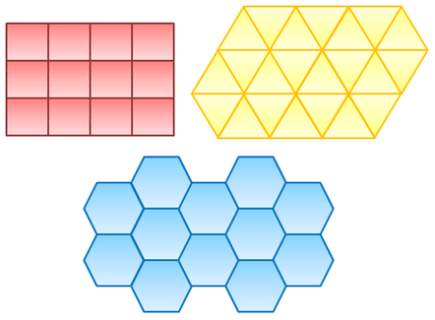Regular tilings of 2D flat space

Hyperbolic 2D surfaces can be partitioned in regular ways in an infinite number of ways! This is because we no longer have the constraints imposed by flat (or spherical) geometries where the angles of shapes must add up to a certain number of degrees. As mentioned, in hyperbolic surfaces the corners of triangles add up to less than 180 degrees, so you can fit more than 6 corners of equilateral triangles at one point (and depending on the curvature of the space, you can fit up to an infinite number of them). Likewise, you can tessellate the entire hyperbolic plane with heptagons.Hyperbolic tiling: Each of the heptagons is just as big (i.e. this is a projection of the real thing)

On the flip side, if you see a regular partitioning of a surface, you can infer what its curvature is! For example, if you see that a surface is entirely covered with heptagons, three on each of the corners, you can be sure that you are seeing a hyperbolic surface. And if you see a surface covered with triangles such that there are only four triangles on each joint, then you know you are seeing a spherical surface. So if you train yourself to notice and count these properties in regular patterns, you will indirectly also be able to determine whether the patterns inhabit a spherical, flat, or hyperbolic space!

### 3) How it applies to experience

How does this apply to experience? Well, in sober states of consciousness one is usually restricted to seeing and imagining spherical and flat surfaces (and their corresponding symmetric partitions). One can of course look at a piece of kale and think “wow, that’s a hyperbolic surface” but what is impossible to do is to see it “as if it were flat”. One can only see hyperbolic surfaces as projections (i.e. where we make regular shapes look irregular so that they can fit on a flat surface) or we end up contorting the surface in a crumbly fashion in order to fit it in our flat experiential space. (Note: even sober phenomenal space happens to be based on projective geometry; but let’s not go there for now.)

### 4) DMT: Hyperbolizing Phenomenal Space

In psychedelic states it is common to experience whatever one looks at (or, with more stunning effects, whatever one hallucinates in a sensorially-deprived environment such as a flotation tank) as slowly becoming more and more symmetric. Symmetrical patterns are attractors in psychedelia. It’s common for people to describe their acid experiences as “a kaleidoscope of colors and meaning”. We should not be too quick to dismiss these descriptions as purely metaphorical. As you can see from the article Algorithmic Reduction of Psychedelic States as well as PsychonautWiki’s Symmetrical Texture Repetition, LSD and other psychedelics do in fact “symmetrify” the textures you experience!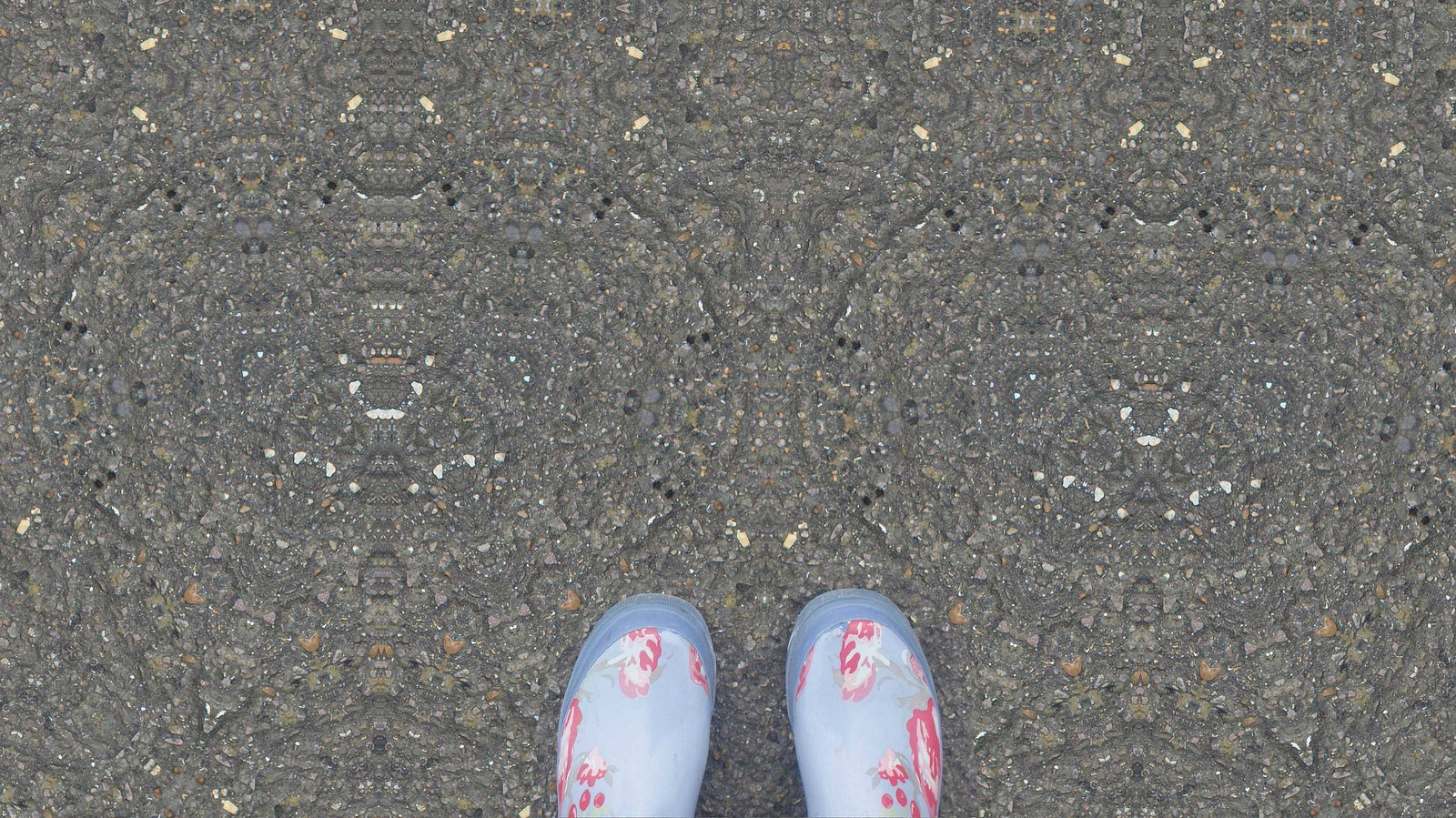What gravel might look like on 150 mics of LSD (Source)

As it turns out, this symmetrification process (what we call “lowering the symmetry detection/propagation threshold”) does allow one to experience any of the possible ways of breaking down spherical and flat surfaces in regular ways (in addition to also enabling the experience of any wallpaper group!). Thus the surfaces of the objects one hallucinates on LSD (specially for Closed Eyes Visuals), are usually carpeted with patterns that have either spherical or flat symmetries (e.g. seeing honeycombs, square grids, regular triangulations, etc.; or seeing dodecahedra, cubes, etc.).17 wallpaper symmetry groups

Only on very high doses of classic psychedelics does one start to experience objects that have hyperbolic curvature. And this is where DMT becomes very relevant. Vaping it is one of the most efficient ways of achieving “unworldly levels of psychedelia”:

On DMT the “symmetry detection threshold” is reduced to such an extent that any surface you look at very quickly gets super-saturated with regular patterns. Since (for reasons we don’t understand) our brain tries to incorporate whatever shape you hallucinate into the scene as part of the scene, the result of seeing too many triangles (or heptagons, or whatever) is that your brain will “push them into the surfaces” and, in effect, turn those surfaces into hyperbolic spaces.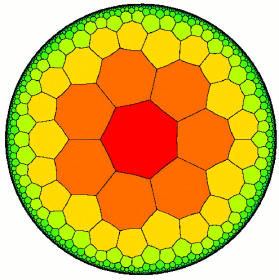Yet another part of your brain (or system of consciousness, whatever it turns out to be) recognizes that “wait, this is waaaay too curved somehow, let me try to shape it into something that could actually exist in my universe”. Hence, in practice, if you take between 10 and 20 mg of DMT, the hyperbolic surfaces you see will become bent and contorted (similar to the pictures you find in the article) just so that they can be “embedded” (a term that roughly means “to fit some object into a space without distorting its properties too much”) into your experience of the space around you.

But then there’s a critical point at which this is no longer possible: Even the most contorted embeddings of the hyperbolic surfaces you experience cannot fit any longer in your normal experience of space on doses above 20 mg, so your mind has no other choice but to change the curvature of the 3D space around you! Thus when you go from “very high on DMT” to “super high on DMT” it feels like you are traveling to an entirely new dimension, where the objects you experience do not fit any longer into the normal world of human experience. They exist in H3 (hyperbolic 3D space). And this is in part why it is so extremely difficult to convey the subjective quality of these experiences. One needs to invoke mathematical notions that are unfamiliar to most people; and even then, when they do understand the math, the raw feeling of changing the damn geometry of your experience is still a lot weirder than you could ever anticipate.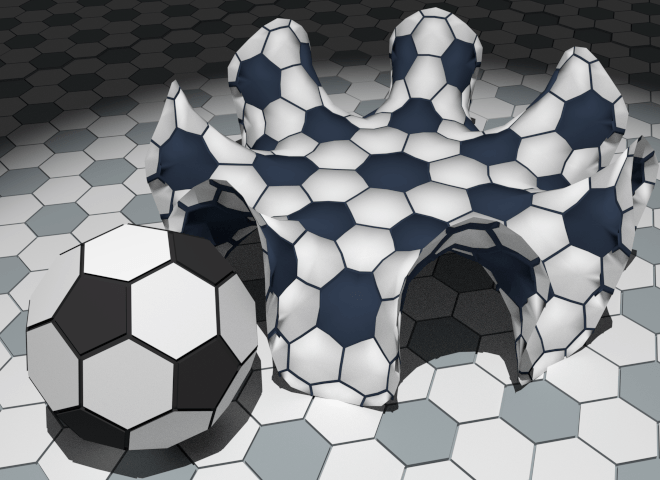Anybody else want to play hyperbolic soccer? Humans vs. Entities, the match of the eon!

### Note: The original article goes into more depth

Now that you understand the gist of the original article, I encourage you to take a closer look at it, as it includes content that I didn’t touch in this ELI5 (or 12) summary. It provides a granular description of the 6 levels of DMT experience (Threshold, Chrysanthemum, Magic Eye, Waiting Room, Breakthrough, and Amnesia), many pictures to illustrate the various levels as well as the particular emergent geometries, and a theoretical discussion of the various algorithmic reductions that might explain how the hyperbolization of phenomenal space takes place based on combining a series of simpler effects together.